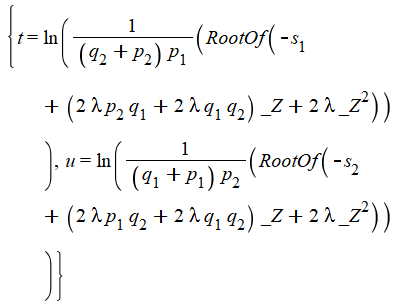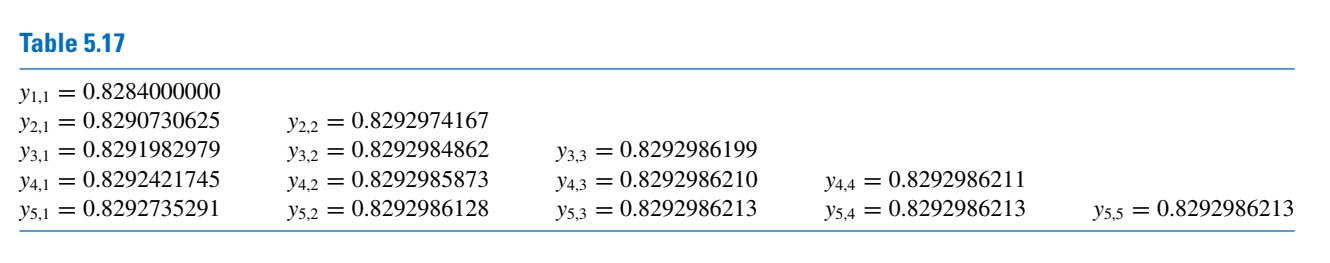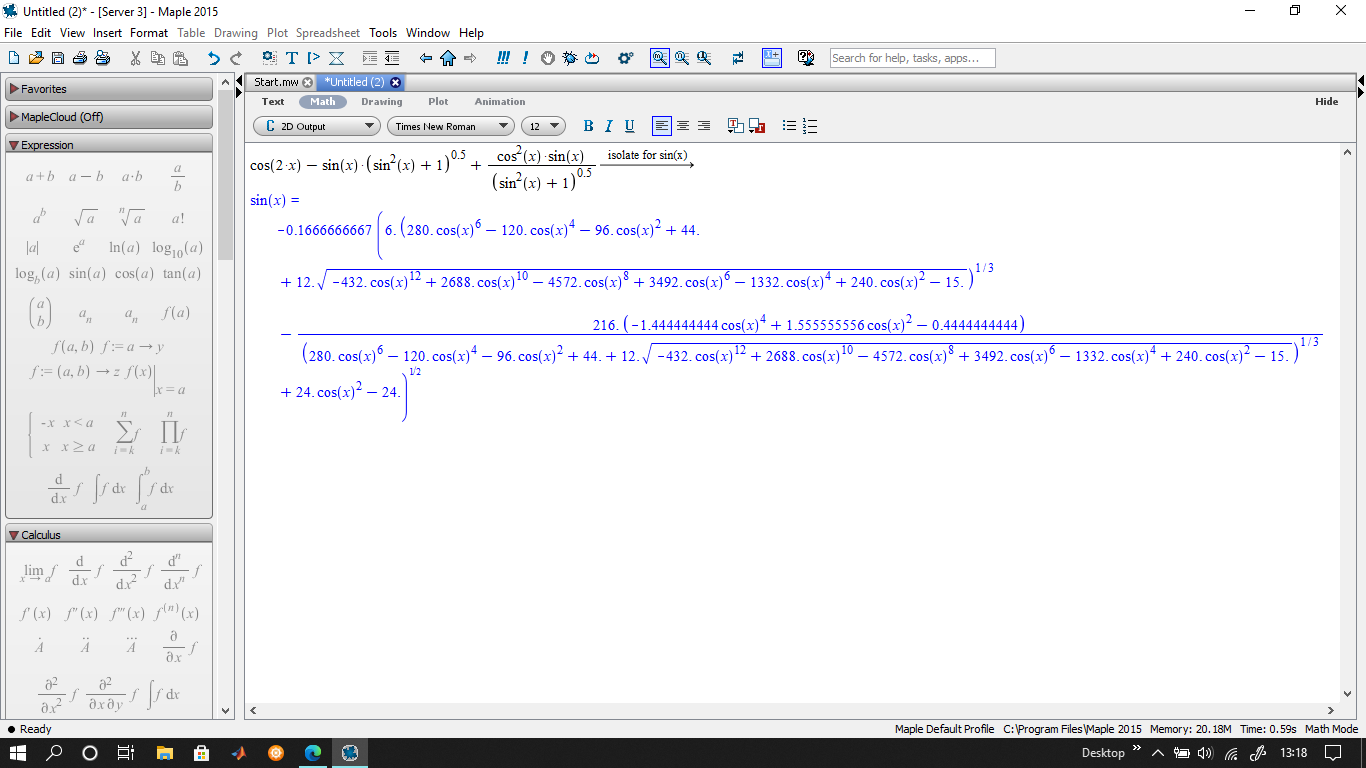## what does _Z and Z_^2 mean?...

I'm solving this equation:

eq3 := 2*lambda*(q+p*exp(t))*(q+p)^2*p*exp(t) = s

eq4 := 2*lambda*(q+p)^2*(q+p*exp(u))*p*exp(u) = s

solve({eq3, eq4}, {t, u})

This is what I get:

{t = ln(RootOf(-s+(2*lambda*p*q+2*lambda*q*q)*_Z+2*lambda*_Z^2)/((q+p)*p)), u = ln(RootOf(-s+(2*lambda*p*q+2*lambda*q*q)*_Z+2*lambda*_Z^2)/((q+p)*p))}I'm completely new at maple.

I can't find what _Z  and _Z^2 mean. Can anybody help? and how I can solve this _z and _Z^2

I've finally decided to see what I could to do with DocumentTools.
I started with these simple lines from ?DocumentTools:-Do

```restart:
with(DocumentTools):
Do(int(%expression, x=%from::integer..%to::integer));
Error, (in DocumentTools:-GetProperty) Attempted to retrieve property of unknown component expression.
```

These next ones return the expected plot but an alert window

```restart:
with(DocumentTools):
Do(%Plot1=plot(x^2, x=0..1));
```However the Click and Drag icon is disabled in the plot toolbar and invisible in the plot menu.

The behaviour of these commands do not depend on whether they are written in a worksheet, a section or a code edit region.

Could you detail me the very first steps do use DocumentTools features?

TIA

DT-Do.mw

## Is there a sequence library available for enumerat...

Is there like https://oeis.org/   Library available in maple for enumerate in for loop?

i would like to like shift 9 times and create 100 mapping for each sequence

## Assign specific name of elements in a Vector...

Hello Users!

I want to assign some specific names of elements in a vector A:

A:=Vector[row](19, {(1) = 14.9057064333276, (2) = 14.4384716751962, (3) = 14.0155569170648, (4) = 13.6381346589334, (5) = 13.3075724008020, (6) = 13.0254476426706, (7) = 12.7935628845392, (8) = 12.6139606264079, (9) = 12.4889383682765, (10) = 12.4210636101451, (11) = 12.4131888520137, (12) = 12.4684665938823, (13) = 12.5903643357509, (14) = 12.7826795776196, (15) = 13.0495548194882, (16) = 13.3954925613568, (17) = 13.8253703032254, (18) = 14.3444555450940, (19) = 14.9584207869626});

like

y[1,1]:=14.9057064333276;

y[1,2]:=14.4384716751962;

y[1,3]:=14.0155569170648;

...

y[1,19]:=14.9584207869626;

Later, I have to use y[1,1], y[1,2],...,y[1,19] for further calculations. I used op command but it does not work. Please help me how I can assign the name.

## Want to generate a table having right triangular f...

Dear Users!

Hope everyone is fine here. I want to formulate the table like give bellow (Table 5.17) in maple so that I can copy it in word file and can edit.The values of y[1,1],y[2,1],y[2,2],y[3,1],y[3,2],y[3,3]...y[nops(HAq),nops(HAq)] present in the following maple code. Thanks in advance

Refine_Extrapolation.mw

## partial differential equations, PDEs...

Hi,

I want to solve this system of PDEs. Please let me know how I can solve it.

Kindly find both my code written in Maple and the image of the problem.

Best,PDE.mw

## Can I make a "library"?...

Is there a way to make a library file that automatically has the functions/procedures loaded on startup and after it any new or open ".mw" can use functions/procedures from library with no redefinition?

## simply sin solution...

It's ok isolate command? I need the solution sin(a)=+-1/(3^0.5)## Is it possible to solve numerically a 2D parabolic...

Let A a (linear) partial differential operator and f=f(x, y, t) a function of space (x, y) and time (t).
Let DXY=[a, b] x [c, d], DT=[0..e] and  dXY the boundary of DXY.
Is it possible, with Maple 2015, to solve numerically the pde Af=0 in the open domain  DXY * DT
with Dirichlet conditions on dXY and initial condition f(DXY, 0)?
It seems that the option 'numeric' of pdsolve doesn't work with more than 1 "non time" independent variable:
Error, (in pdsolve/numeric/process_PDEs) can only numerically solve PDE with two independent variables, got {t, x, y}

Nevertheles I seem to remember seeing this on Mapleprime (?)
If Maple 2015 can't do it, can Maple 2020?

• Remark: Af=0 is the heat equation with rho=Cp=lambda=1.
I guess I could code the alternate directions method to transform  Af=0 into a sequence Axf=0,  Ayf=0 Axf=0 ... of 1D diffusion equations but I'm a little bit lazzy and I'm waiting for your feedback before doing this.

## Collocating a vector to make a square matrix...

Dear Users!

Hope everyone fine here. I want to collocate Vector IntXYZ1 and IntXYZ2 present in file Q1 in such a way that the first M1M2 + 2(M3-1)M2 + 2(M3-1)(M1-2) rows are zero and other rows are collocated at x=(i-1)/(M1-1), y=(j-1)/(M2-1), t=(k-1)/(M3-1) for i = 2,3,…,M1-1, j = 2,3,…,M2-1, k = 2,3,…,M3 as given as XX (of order 27 by 27 with first 25 rows are zero) for M1=M2=M3=3I am waiting for positive response to fix this issue. Thanks in advance

Q1.mw

## Why is "global" only allowed in procedures?...

Hi,

Why does the use of  global  at the top level of a worksheet is no longer accepted in the most recent versions of Maple (it still was in Maple 2015), but only within procedures?

TIA

## How to add a legend to an histogram?...

Hi

How to add a legend to Statistics:-Histogram?

This could be particularly useful to compare histograms, for instance in

```S := Statistics:-Sample(Normal(0, 1), 1000):
T := Statistics:-Sample(Normal(1, 1), 1000):

plots:-display(
Statistics:-Histogram(S, color=gold, transparency=0.3, legend="N(0,1)"),
Statistics:-Histogram(T, transparency=0.3, legend="N(1,1)")
);
```

But doing this generates the error Error, (in Statistics:-Histogram) display does not accept the legend option

And yes indeed, even this command

`h := Statistics:-Histogram(S, legend="N(0,1)")`

After having examined the structure of h and using a way Carl Love gave here How to change the colour inn Statistics:-Sunflower?  I finally obtained the expected plot.

```HS := Statistics:-Histogram(S, color=gold, transparency=0.3):
HT := Statistics:-Histogram(T, transparency=0.3):

hs := evalindets([op(HS)], specfunc(COLOUR), c-> (c, LEGEND("N(0,1)")) ):
ht := evalindets([op(HT)], specfunc(COLOUR), c-> (c, LEGEND("N(1,1)")) ):

PLOT(op(hs), op(ht))
```But this rather long a way to proceed (Note: it seems that the overrideoption  that acer suggested in this prevous thread doesn't "work" here?)
Do you have any idea if there is a simpler solution?

TIA

## Transformation of a vector...

Dear Users!
Hope everyone fine here. I have a vector V and want a new vector after transformation like

V := Vector(5, {(1) = 2, (2) = 3, (3) = -1, (4) = 9, (5) = 7});

after square transformation of V I got a new vector W like bellow

W := Vector(5, {(1) = 4, (2) = 9, (3) = 1, (4) = 81, (5) = 49});

and similarly sin transformation of V give the following matrix as

U := Vector(5, {(1) = sin(2), (2) = sin(3), (3) = sin(-1), (4) = sin(9), (5) = sin(7)});

## Need to reduce the Jacobian matrix construction as...

Dear Users!
Hope everyone fine here. I tried (as given bellow) to find the solution of nonlinear system of PDEs via FDM. To solve system of nonlinear equations I used newton raphson method and for higher value of like Mx > 8 the matrix G and G1 (mentioned as red) take alot of time. Can anyone help me to reduce the computational time? Becuase I have to evealuate the solution for Mx = 50.

restart; Digits := 30; with(LinearAlgebra);
T := 1; L := 3; N := 30; Mx := 5; `&Delta;x` := L/(1.*Mx); `&Delta;t` := T/(1.*N);
for i from 0 while i <= Mx do
u[i, 0] := 0.; u[i, -1] := u[i, 1]; tau[i, 0] := 0.; theta[i, 0] := 0.; theta[i, -1] := theta[i, 1]
end do;
for n from 0 while n <= N do u[0, n] := 0.; u[Mx, n] := 0.; theta[0, n] := 1.; theta[Mx, n] := 0.
end do;
for n from 0 while n <= N-1 do
print("Simulation in proccess at time-level n", n+1);
for i while i <= Mx-1 do
Ru[i, n] := simplify((u[i+1, n+1]-u[i+1, n])/`&Delta;t`+(u[i+1, n+1]-2*u[i+1, n]+u[i+1, n-1])/`&Delta;t`^2-(u[i+1, n+1]-2*u[i, n+1]+u[i-1, n+1])/`&Delta;x`^2+25.*(u[i+1, n+1]+(u[i+1, n+1]-u[i+1, n])/`&Delta;t`)-1.5*(theta[i, n]+(theta[i, n+1]-theta[i, n])/`&Delta;t`));
`R&theta;`[i, n] := simplify((theta[i+1, n+1]-theta[i+1, n])/`&Delta;t`+(theta[i+1, n+1]-2*theta[i+1, n]+theta[i+1, n-1])/`&Delta;t`^2-(theta[i+1, n+1]-2*theta[i, n+1]+theta[i-1, n+1])/((15.)*`&Delta;x`^2)-((u[i, n+1]-u[i-1, n+1])/`&Delta;x`)^2/(3.)) end do;
for i while i <= Mx-1 do
`R&tau;`[i, n] := simplify(tau[i+1, n+1]+(tau[i+1, n+1]-tau[i+1, n])/`&Delta;t`-1.5^(-1/4)*(u[i+1, n+1]-u[i, n+1])/`&Delta;x`)
end do;
Sys := `<,>`(seq(Ru[i, n], i = 1 .. Mx-1), seq(`R&tau;`[i, n], i = 1 .. Mx-1), seq(`R&theta;`[i, n], i = 1 .. Mx-1));
V := `<,>`(seq(u[i, n+1], i = 1 .. Mx-1), seq(theta[i, n+1], i = 1 .. Mx-1), seq(tau[i, n+1], i = 2 .. Mx));
G := Matrix(3*(Mx-1), proc (i, j) options operator, arrow; diff(Sys[i], V[j]) end proc); G1 := MatrixInverse(G);
X[n, 0] := Vector(1 .. 3*(Mx-1), 1);
for k1 from 0 to r do
X[n, k1+1] := eval(V-G1 . Sys, Equate(V, X[n, k1]))
end do;
Sol[n] := Equate(V, X[n, r+1]); assign(op(Sol[n]));
if n > 0 then
U := eval(`<,>`(seq(u[i1, n+1], i1 = 1 .. Mx)-seq(u[i1, n], i1 = 1 .. Mx))); Noru[n+1] := Norm(%, 2); print("L[&infin;] norm of &tau;(x,y,t) at time level = ", %);
Theta := eval(`<,>`(seq(theta[i1, n+1], i1 = 0 .. Mx)-seq(theta[i1, n], i1 = 0 .. Mx))); `Nor&theta;`[n+1] := Norm(%, 2); print("L[&infin;] norm of &theta;(x,y,t) at time level = ", %) else print("n < 0")
end if end do

Special request to:
@acer @Carl Love @Kitonum @Preben Alsholm

## Numerical solution of system PDEs...

Dear Users!

I want to find the solution of the solution of PDEs as given bellow:

restart;
PDE1 := diff(u(y, t), t)+diff(u(y, t), t, t) = diff(u(y, t), y, y)-u(y, t);
PDE2 := v(y, t)+diff(tau(y, t), t) = diff(u(y, t), y);
ICandBC := {tau(y, 0) = 0, u(0, t) = 0, u(3, t) = 0, u(y, 0) = 0, (D(u))(y, 0) = 0};
pds := pdsolve({PDE1, PDE2}, ICandBC, numeric);

But got the following error

Error, (in pdsolve/numeric/process_PDEs) number of dependent variables and number of PDE must be the same
Kindly help me to fix this error. I shall be very thankful.

Special request to:
@acer @Carl Love @Kitonum @Preben Alsholm

﻿